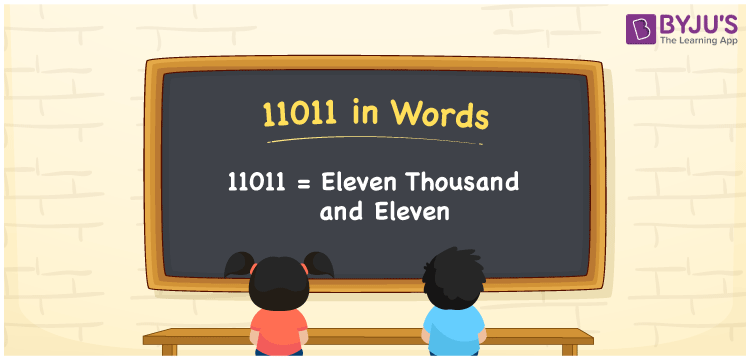# 11011 in Words

The numeral 11011 in words is “Eleven thousand and eleven”. The number 11011 appears after the number 11010 and before the number 11012. Also, the number 11011 is a cardinal number. For example, Meera purchased a mobile worth Rs. 11011. It can also be written as “Meera purchased a mobile worth Rs. Eleven thousand and eleven.

 11011 in Words: Eleven Thousand and Eleven. Eleven Thousand and Eleven in Numerical Form: 11011.

## 11011 in English Words## How to Write 11011 in Words?

Learn the place values of 11011 up to 5 digits using the below table.

 Ten-thousands Thousands Hundreds Tens Ones 1 1 0 1 1

The expanded form of 11011 is as follows:

= 1 × Ten thousand + 1 × Thousand + 0 × Hundred + 1 × Ten + 1 × One

= 1 × 10000 + 1 × 1000 + 0 × 100 + 1 × 10 + 1 × 1

= 10000 + 1000 + 10 + 1

= 11011

= Eleven thousand and eleven

Hence, 11011 in words is eleven thousand and eleven.

11011 in words – Eleven thousand and eleven

Is 11011 an odd number? – Yes

Is 11011 an even number? – No

Is 11011 a perfect square number? – No

Is 11011 a perfect cube number? – No

Is 11011 a prime number? – No

Is 11011 a composite number? – Yes

## Frequently Asked Questions on 11011 in Words

Q1

### How to write 11011 in words?

11011 in words is eleven thousand and eleven.

Q2

### Simplify 11000 + 11, and express it in words.

Simplifying 11000 + 11, we get 11011. Hence, 11011 in words is eleven thousand and eleven.

Q3

### Is 11011 an odd number?

Yes, 11011 is an odd number.